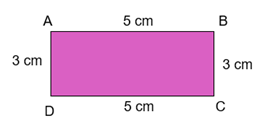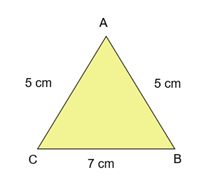# What is Perimeter in Maths | Find the Perimeter of Shapes

What is Perimeter in Maths?

Perimeter is the sum of all the sides of a closed figure.

Perimeter = Sum of all the sides

Find the Perimeter of Shapes

Example 1

Find the Perimeter of the Rectangle:Solution

Perimeter is the sum of all the sides of a closed figure.

Perimeter = Sum of all the sides

Perimeter of the given rectangle = AB + BC + CD + DA

Perimeter of the given rectangle = 5 cm + 3 cm + 5 cm + 3 cm

Perimeter of the given rectangle = 16 cm

Example 2

Find the Perimeter of the triangle:Solution

Perimeter is the sum of all the sides of a closed figure.

Perimeter = Sum of all the sides

Perimeter of the given Triangle = AB + BC + CA

Perimeter of the given Triangle = 5 cm + 7 cm + 5 cm

Perimeter of the given Triangle = 17 cm